# TSA Covariance VI

LabVIEW 2014 Advanced Signal Processing Toolkit Help

Edition Date: June 2014

Part Number: 372656C-01

»View Product InfoDownload Help (Windows Only)

Owning Palette: Statistical Analysis VIs

Computes the covariance matrix or correlation matrix of a multivariate (vector) time series. Wire data to the Xt input to determine the polymorphic instance to use or manually select the instance.

Use the pull-down menu to select an instance of this VI.

 Select an instance TSA Covariance (Waveform)TSA Covariance (Array)

## TSA Covariance (Waveform)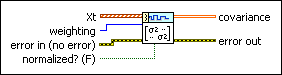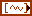Xt specifies the multivariate (vector) time series.weighting specifies which variance to calculate. Options include Sample and Population. The default is Sample (N–1). Refer to the Details section for information about how this parameter affects the variance value.error in describes error conditions that occur before this node runs. This input provides standard error in functionality.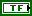normalized? specifies to normalize the results into a covariance matrix, where the nondiagonal elements are close to one. The default is FALSE.covariance returns the calculated covariance matrix of the multivariate (vector) time series.error out contains error information. This output provides standard error out functionality.

## TSA Covariance (Array)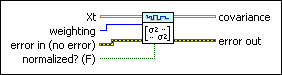Xt specifies the multivariate (vector) time series. Each column of the 2D array represents a vector at certain time.weighting specifies which variance to calculate. Options include Sample and Population. The default is Sample (N–1). Refer to the Details section for information about how this parameter affects the variance value.error in describes error conditions that occur before this node runs. This input provides standard error in functionality.normalized? specifies to normalize the results into a covariance matrix, where the nondiagonal elements are close to one. The default is FALSE.covariance returns the calculated covariance matrix of the multivariate (vector) time series.error out contains error information. This output provides standard error out functionality.

## TSA Covariance Details

When normalized? is FALSE, this VI calculates the covariance matrix for a multivariate time series m according to the following equation: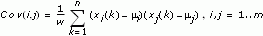xi, as a row vector, is the ith channel time series. mi is the arithmetic mean of xi. The dimension of the covariance matrix is m×m. w is weighting. w=n when weighting is set to Population. w=n–1 when weighting is set to Sample.

When normalized? is TRUE, this VI calculates the correlation coefficient matrix according to the following equation: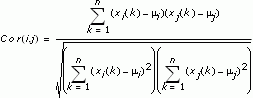The above operation is equivalent to unifying each channel xi with zero mean and unit energy and then calculating the covariance matrix of the unified multivariate time series.